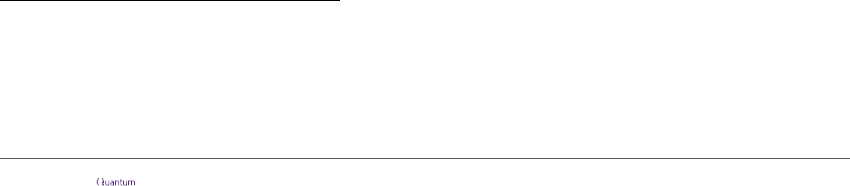Graph-theoretic Simpliﬁcation of Quantum Circuits
with the ZX-calculus
Ross Duncan
1,2
, Aleks Kissinger
3
, Simon Perdrix
4
, and John van de Wetering
5
1
University of Strathclyde, 26 Richmond Street, Glasgow G1 1XH, UK
2
Cambridge Quantum Computing Ltd, 9a Bridge Street, Cambridge CB2 1UB, UK
3
Department of Computer Science, University of Oxford
4
CNRS LORIA, Inria-MOCQUA, Université de Lorraine, F 54000 Nancy, France
5
Institute for Computing and Information Sciences, Radb oud University Nijmegen
May 27, 2020
We present a completely new approach to quantum circuit optimisation,
based on the ZX-calculus. We ﬁrst interpret quantum circuits as ZX-diagrams,
which provide a ﬂexible, lower-level language for describing quantum compu-
tations graphically. Then, using the rules of the ZX-calculus, we give a simpli-
ﬁcation strategy for ZX-diagrams based on the two graph transformations of
local complementation and pivoting and show that the resulting reduced dia-
gram can be transformed back into a quantum circuit. While little is known
about extracting circuits from arbitrary ZX-diagrams, we show that the under-
lying graph of our simpliﬁed ZX-diagram always has a graph-theoretic property
called generalised ﬂow, which in turn yields a deterministic circuit extraction
procedure. For Cliﬀord circuits, this extraction procedure yields a new normal
form that is both asymptotically optimal in size and gives a new, smaller up-
per bound on gate depth for nearest-neighbour architectures. For Cliﬀord+T
and more general circuits, our technique enables us to to ‘see around’ gates
that obstruct the Cliﬀord structure and produce smaller circuits than naïve
‘cut-and-resynthesise’ methods.
1 Introduction
Quantum circuits provide a de facto ‘assembly language’ for quantum computation, in
which computations are described as the composition of many simple unitary linear maps
called quantum gates. An important topic in the study of quantum circuits is quantum
circuit optimisation, whereby quantum circuits which realise a given computation are trans-
formed into new circuits involving fewer or simpler gates. While some strides have already
been made in this area, the ﬁeld is still relatively undeveloped. Most approaches to quan-
tum circuit optimisation are based on only a handful of techniques: gate substitutions,
computation of small (pseudo-)normal forms for special families of circuits [1, 29, 32],
optimisation of phase polynomials [3, 22], or some combination thereof [2, 35].
Ross Duncan: ross.duncan@strath.ac.uk
Aleks Kissinger: aleks.kissinger@cs.ox.ac.uk
Simon Perdrix: simon.perdrix@loria.fr
John van de Wetering: john@vdwetering.name
Accepted in Quantum 2020-04-27, click title to verify 1
arXiv:1902.03178v6 [quant-ph] 26 May 2020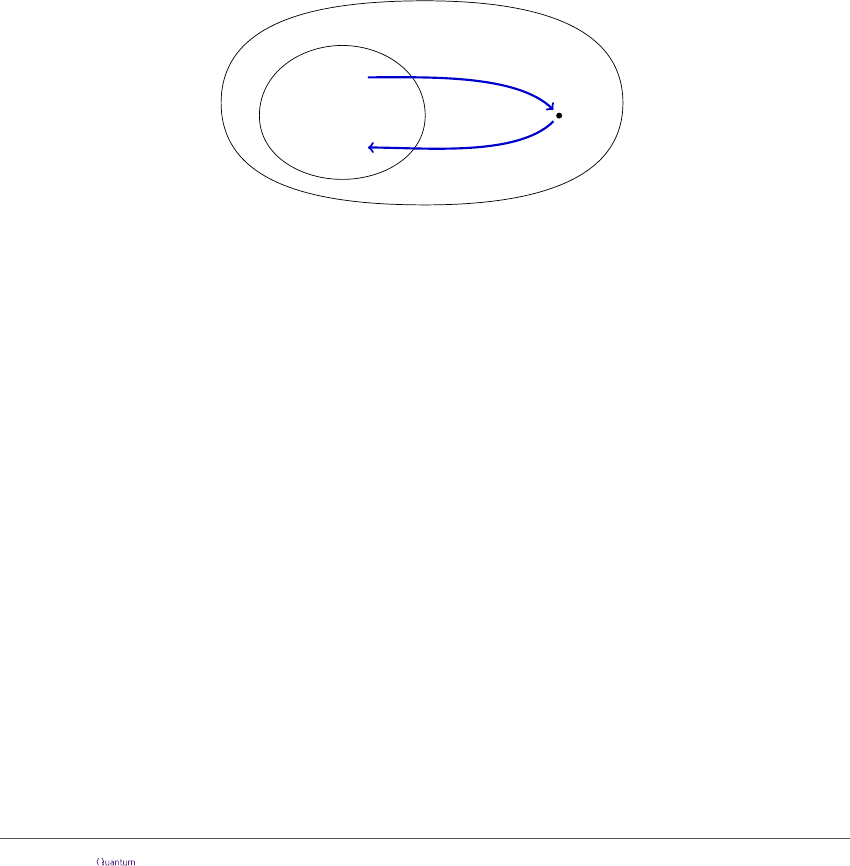This paper lays a theoretical foundation for a completely new quantum circuit optimi-
sation technique based on the ZX-calculus . A key point of departure of our technique
is that we break the rigid structure of quantum circuits and perform reductions on a lower-
level, string diagram-based representation of a quantum circuit called a ZX-diagram. These
diagrams are more ﬂexible than circuits, in that they can be deformed arbitrarily, and are
subject to a rich equational theory: the ZX-calculus. The core rules of the ZX-calculus
give a sound and complete  theory for Cliﬀord circuits, a well-known class of circuits
that can be eﬃciently classically simulated. More surprisingly, it was shown in 2018 that
modest extensions to the ZX-calculus suﬃce to give completeness for families of circuits
that are approximately universal [24, 25] and exactly universal [9, 20, 23, 39] for quantum
computation.
Since ZX-diagrams provide a more ﬂexible representation of a quantum computation
than a circuit, we can derive simpliﬁcations of ZX-diagrams that have no quantum circuit
analogue. However, this added ﬂexibility comes at a price: while any quantum circuit can
be interpreted as a ZX-diagram by decomposing gates into smaller pieces, the converse is
not true. For a generic ZX-diagram corresponding to a unitary, there is no known general-
purpose procedure for eﬃciently recovering a quantum circuit. Hence, an important part
of our optimisation procedure is keeping enough information about the quantum circuit
structure to get a circuit back at the end. Schematically:
ZX-diagrams
Quantum circuits
simpliﬁcation
circuit extraction
Until recently, this extraction step was poorly understood, and the only techniques
for doing circuit-to-circuit translation with the ZX-calculus did so without departing from
the overall structure of the original quantum circuit , avoiding the extraction problem
altogether. In this paper we adopt a more ambitious approach. First, building on prior
work of two of the authors , we use the rules of the ZX-calculus to derive a sound,
terminating simpliﬁcation procedure for ZX-diagrams. The key simpliﬁcation steps involve
the graph-theoretic transformations of local complementation and pivoting [6, 30], which
allow certain generators to be deleted from ZX-diagrams one-by-one or in pairs, respec-
tively. When applied to Cliﬀord circuits, the diagram resulting from the simpliﬁcation
procedure is represented in the form of a graph-state with local Cliﬀords (GS-LC) , a
pseudo-normal form for representing Cliﬀord circuits whose size is at most quadratic in
the number of qubits. Hence, one side eﬀect of our simpliﬁcation procedure is that it gives
a simple, graph-theoretic alternative to the normalisation of a ZX-diagram to GS-LC form
proposed by Backens . For non-Cliﬀord circuits, the simpliﬁed ZX-diagram represents
a ‘skeleton’ of the circuit we started with, consisting only of generators arising from non-
Cliﬀord phase gates and their nearest neighbours. Although this is no longer a canonical
form, it can still be signiﬁcantly smaller than the input circuit, especially when there is
a relatively low proportion of non-Cliﬀord gates. We then show that, even though this
simpliﬁcation breaks the circuit structure, it preserves a graph-theoretic invariant called
focused gFlow [8, 34], from which we can derive an eﬃcient circuit extraction strategy. We
demonstrate the full simplify-and-extract procedure by means of a running example, which
Accepted in Quantum 2020-04-27, click title to verify 2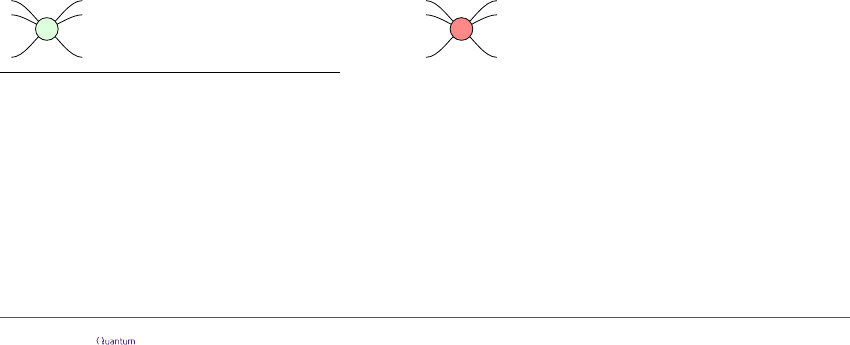is also available online as a Jupyter notebook.
1
In the case of Cliﬀord circuits, this procedure will produce circuits comparable in size
to those described by standard techniques [1, 38]. In the non-Cliﬀord case, this can already
ﬁnd more simpliﬁcations than naïvely ‘cutting’ the circuit and simplifying purely-Cliﬀord
sections. More importantly, this paper establishes a new theoretical framework upon which
to build powerful new optimisation techniques, e.g. for important tasks such as T-count
reduction. Indeed an ancilla-free T-count reduction technique based on the framework
of this paper has recently been introduced in Ref.  and implemented in a tool called
PyZX . At the time of publication, this new technique matched or out-performed the
state of the art on 72% of the benchmark circuits tested, in some cases decreasing the
T-count by as much as 50%.
2
The paper is organised as follows. After giving a brief introduction to the ZX-calculus
in Section 2, we will introduce graph-like ZX-diagrams in Section 3. This mild restriction
to the family of all ZX-diagrams will enable us to speak more readily about graph-theoretic
properties of the diagram, like the existence of a focused gFlow. In Section 4 we will show
that both local complementation and pivoting preserve focused gFlow. Then in Section 5
we will derive a simpliﬁcation routine using these graph-theoretic notions. In Section 6
we study the properties of Cliﬀord diagrams resulting from this simpliﬁcation routine,
and show how these can be transformed into circuits. Then in Section 7 we show how
general diagrams produced by this routine can be extracted into a circuit, and give some
experimental evidence that this method performs better than only optimizing Cliﬀord
sub-circuits. Finally, in Section 8 we conclude and discuss future work.
Note: The results in Proposition 6.1 concerning Cliﬀord gate count and depth were added
to a version of this paper submitted for peer review in October 2019. Prior to us making
this version public, Bravyi and Maslov independently reported similar results in Ref. .
2 The ZX-calculus and quantum circuits
We will provide a brief overview of the ZX-calculus. For an in-depth reference see Ref. .
The ZX-calculus is a diagrammatic language similar to the familiar quantum circuit
notation. A ZX-diagram consists of wires and spiders. Wires entering the diagram from
the left are inputs; wires exiting to the right are outputs. Given two diagrams we can
compose them by joining the outputs of the ﬁrst to the inputs of the second, or form their
tensor product by simply stacking the two diagrams.
Spiders are linear maps which can have any number of input or output wires. There
are two varieties: Z spiders depicted as green dots and X spiders depicted as red dots.
3
Written explicitly in Dirac notation, these linear maps are:
α
...
...
:= |0...0ih0...0| + e
|1...1ih1...1|
α
...
...
:= |+...+ih+...+| + e
|−...−ih−...−|
1
nbviewer.jupyter.org/github/Quantomatic/pyzx/blob/906f6db3/demos/example-gtsimp.ipynb
(with PyZX installed). The simplest way to do this is to clone or download the PyZX repostory from:
github.com/quantomatic/pyzx and ﬁnd the notebook in demos.
2
The ‘PyZX-only’ T-counts from  were subsequently matched by parallel, independent work of
Zhang and Chen .
3
If you are reading this document in monochrome or otherwise have diﬃculty distinguishing green and
Accepted in Quantum 2020-04-27, click title to verify 3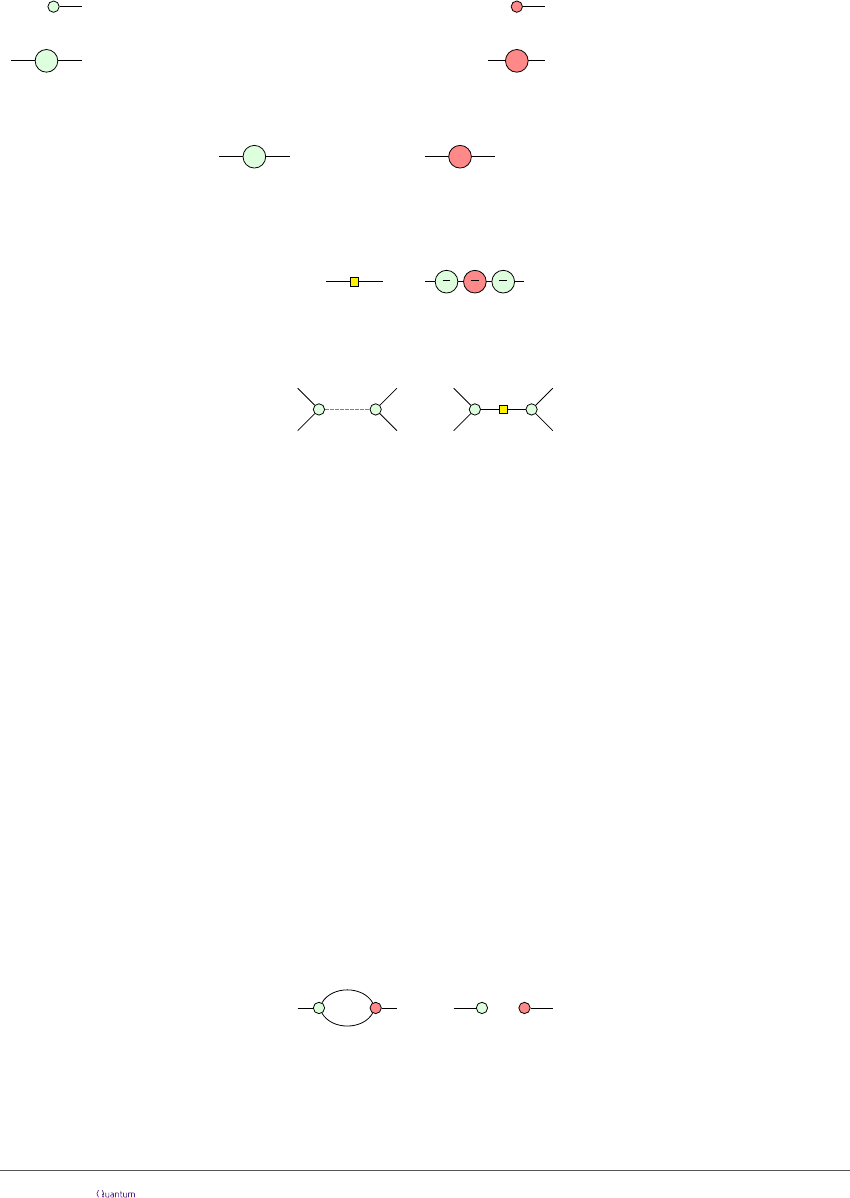Therefore a ZX-diagram with m input wires and n output wires represents a linear map
(C
2
)
m
(C
2
)
n
built from spiders (and permutations of qubits) by composition and
tensor product of linear maps. As a special case, diagrams with no inputs and n outputs
represent vectors in (C
2
)
n
, i.e. (unnormalised) n-qubit states.
Example 2.1. We can immediately write down some simple state preparations and uni-
taries in the ZX-calculus:
= |0i + |1i |+i = |+i + |−i |0i
α
= |0ih0| + e
|1ih1| = Z
α
α
= |+ih+| + e
|−ih−| = X
α
In particular we have the Pauli matrices:
π
= Z
π
= X
It will be convenient to introduce a symbol a yellow square for the Hadamard gate.
This is deﬁned by the equation:
=
π
2
π
2
π
2
(1)
We will often use an alternative notation to simplify the diagrams, and replace a Hadamard
between two spiders by a blue dashed edge, as illustrated below.
:=
...
...
...
...
Both the blue edge notation and the Hadamard box can always be translated back into
spiders when necessary. We will refer to the blue edge as a Hadamard edge.
Two diagrams are considered equal when one can be deformed to the other by moving
the vertices around in the plane, bending, unbending, crossing, and uncrossing wires, so
long as the connectivity and the order of the inputs and outputs is maintained. Further-
more, there is an additional set of equations that we call the rules of the ZX-calculus; these
are shown in Figure 1.
Remark 2.2. We neglect (non-zero) scalar factors in the rules in Figure 1. That is, if
we are able to prove two ZX-diagrams are equal using the rules of Figure 1, then their
associated matrices satisfy A = zB for z C\{0}. It is possible to give a presentation
of the ZX-calculus that accounts for scalar factors (see e.g. ), but for our purposes
it will not be necessary. This is because the inputs and outputs of our simpliﬁcation
procedure are quantum circuits, which are unitary by construction. If A and B are unitary,
A = zB = |z| = 1, so neglecting scalars will (at worst) produce and output that diﬀers
from the input by a global phase.
Let us derive two additional rules, known as the antipode rule and the π-copy rule.
Lemma 2.3. The antipode rule,
=
(a)
is derivable in the ZX-calculus.
Proof. To derive the (a) rule we take advantage of the freedom to deform the diagram as
shown below.
Accepted in Quantum 2020-04-27, click title to verify 4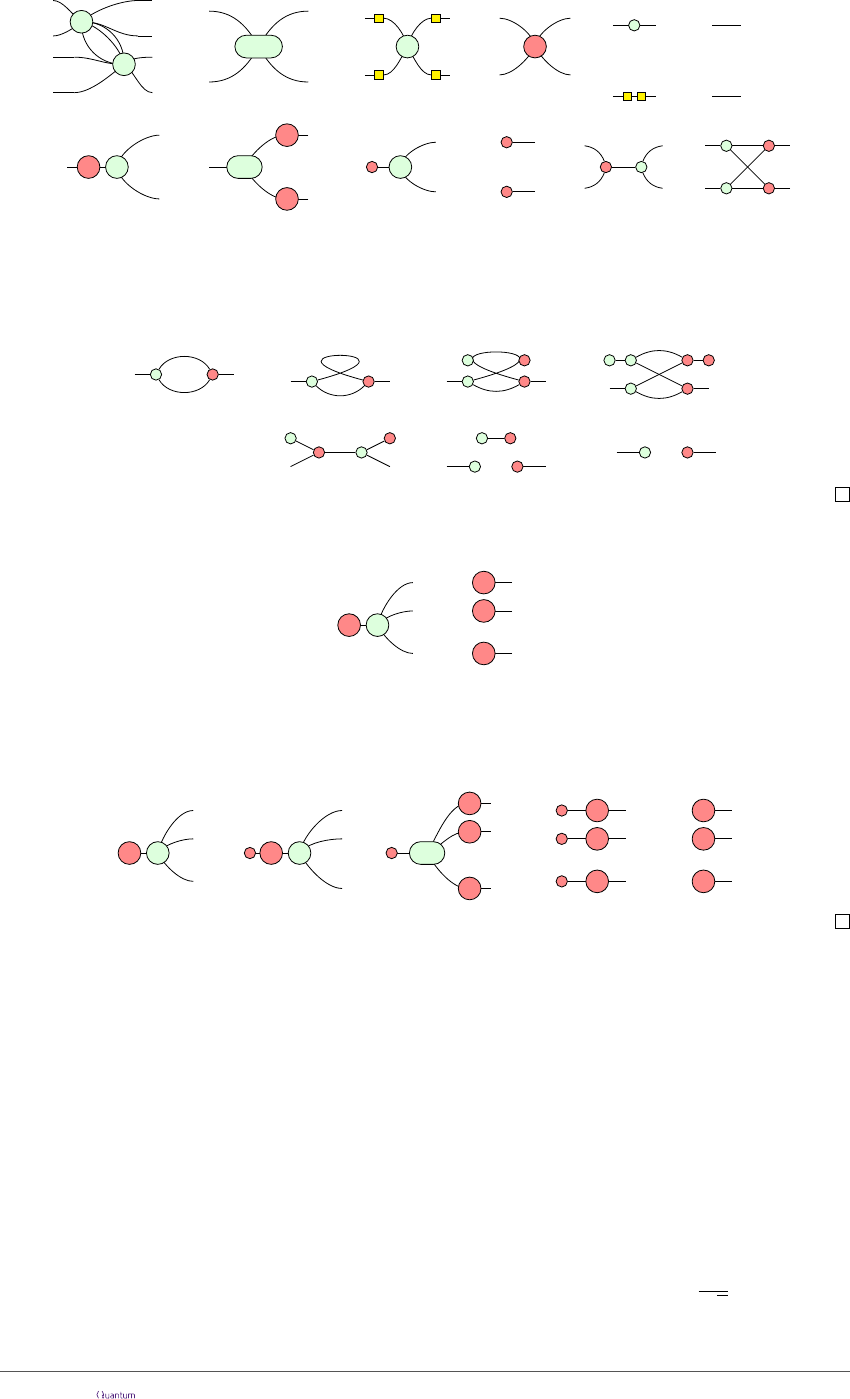β
...
...
α
...
...
=
...
...
...
α+β
(f)
α
=
π
π α
...
...
π
(π)
...
α
=
...
(c)
...
=
...
(h)
(i1)
=
=
(i2)
(b)
=
...
α α
...
Figure 1: A convenient presentation for the ZX-calculus. These rules hold for all α, β [0, 2π), and
due to (h) and (i2) all rules also hold with the colours interchanged. Note ... should be read as ‘0 or
more’, hence the spiders on the LHS of (f ) are connected by one or more wires.
==
=
=
= =
(i1) (f)
(b) (c)
The ﬁnal equation is simply dropping the scalar.
Lemma 2.4. The π-copy rule,
π
=
...
(πc)
π α
π
...
π
is derivable in the ZX-calculus.
Proof. To derive the (πc) rule we use the (f ) rule ﬁrst to split the π-labelled vertex, and
the (π) and (c) rules do the bulk of the work as shown below:
π
=
...
π α
π
...
π
π
...
...
π
π
(π)
α
α
π
=
(f)
π
π
π
...
(f)
=
(c)
=
Remark 2.5. The ZX-calculus is universal in the sense that any linear map can be
expressed as a ZX-diagram. Furthermore, when restricted to Cliﬀord ZX-diagrams, i.e.
diagrams whose phases are all multiples of π/2, the version we present in Figure 1 is
complete. That is, for any two Cliﬀord ZX-diagrams that describe the same linear map,
there exists a series of rewrites transforming one into the other. Recent extensions to
the calculus have been introduced which are complete for the larger Cliﬀord+T family of
ZX-diagrams , where phases are multiples of π/4, and for all ZX-diagrams [20, 23, 39].
Quantum circuits can be translated into ZX-diagrams in a straightforward manner. We
will take as our starting point circuits constructed from the following universal set of gates:
CNOT :=
1 0 0 0
0 1 0 0
0 0 0 1
0 0 1 0
Z
α
:=
1 0
0 e
!
H :=
1
2
1 1
1 1
!
Accepted in Quantum 2020-04-27, click title to verify 5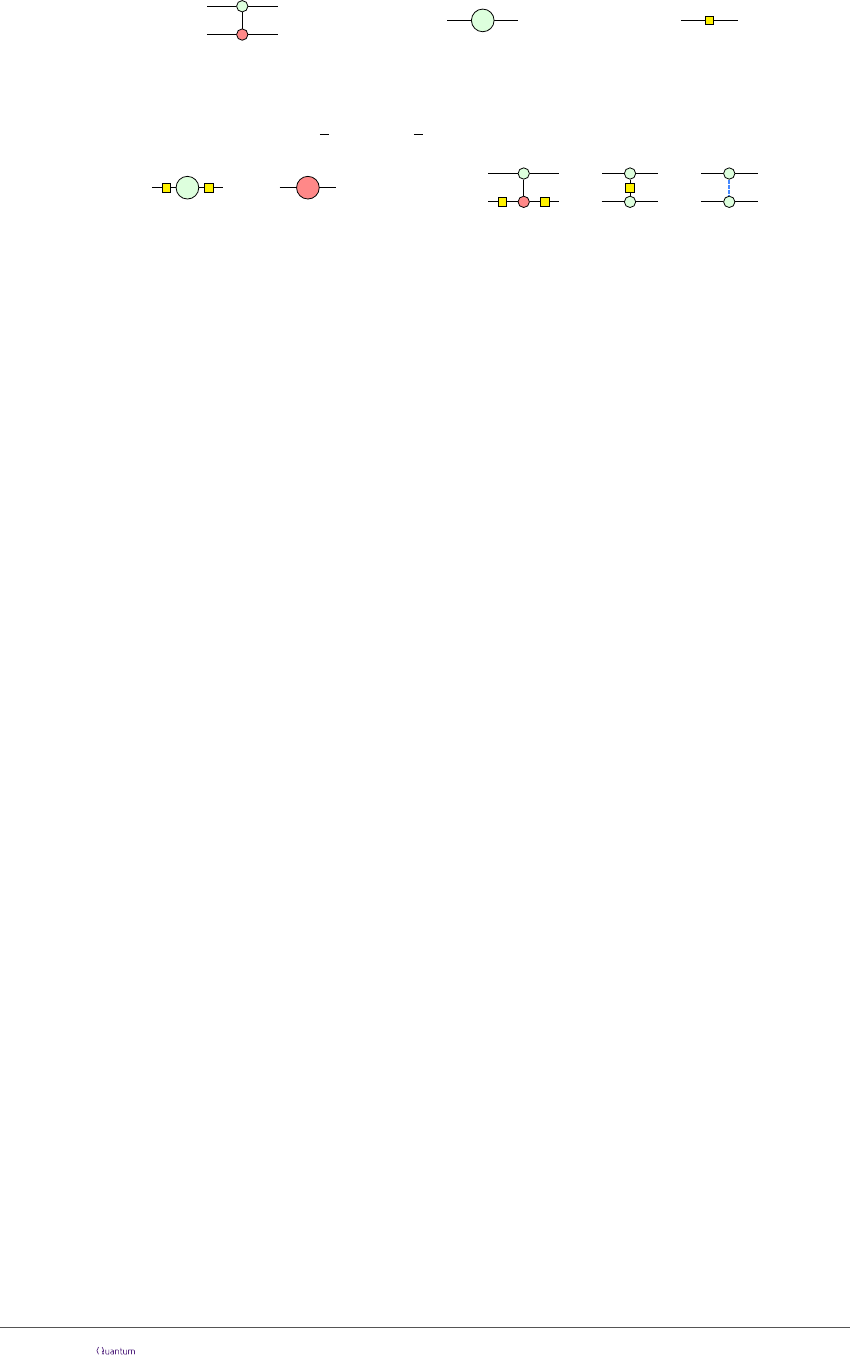We ﬁx this gate set because they admit a convenient representation in terms of spiders:
CNOT = Z
α
=
α
H = (2)
For the CNOT gate, the green spider is the ﬁrst (i.e. control) qubit and the red spider is
the second (i.e. target) qubit. Other common gates can easily be expressed in terms of
these gates. In particular, S := Z
π
2
, T := Z
π
4
and:
X
α
=
αα
=
CZ =
= =
Remark 2.6. Note that the directions of the wires in the depictions of the CNOT and
CZ gates are irrelevant, hence we can draw them vertically without ambiguity. This
undirectedness of wires is a general property of ZX-diagrams, and from hence forth we will
ignore wire directions without further comment. We will also freely draw wires entering
or exiting the diagram from arbitrary directions if the interpretation (i.e. as an input or
an output) is either irrelevant or clear from context.
For our purposes, we will deﬁne quantum circuits as a special case of ZX-diagrams.
Deﬁnition 2.7. A circuit is a ZX-diagram generated by compositions and tensor products
of the ZX-diagrams in equation (2).
Important subclasses of circuits are Cliﬀord circuits, sometimes called stabiliser circuits,
which are obtained from compositions of only CNOT, H, and S gates. They are eﬃciently
classically simulable, thanks to the Gottesman-Knill theorem . A unitary is local Cliﬀord
if it arises from a single-qubit Cliﬀord circuit, i.e. a composition of H and S gates. The
addition of T gates yields Cliﬀord+T circuits, which are capable of approximating any
n-qubit unitary to arbitrary precision, whereas the inclusion of Z
α
gates for all α enables
one to construct any unitary exactly .
3 Graph-like ZX-diagrams
Before starting our simpliﬁcation procedure, we transform ZX-diagrams into the following,
convenient form.
Deﬁnition 3.1. A ZX-diagram is graph-like when:
1. All spiders are Z-spiders.
2. Z-spiders are only connected via Hadamard edges.
3. There are no parallel Hadamard edges or self-loops.
4. Every input or output is connected to a Z-spider and every Z-spider is connected to
at most one input or output.
A ZX-diagram is called a graph state if it is graph-like, every spider is connected to
an output, and there are no non-zero phases. This corresponds to the standard notion of
graph state from the literature . Hence, graph-like ZX-diagrams are a generalisation
of graph states to maps where we allow arbitrary phases and some interior spiders, i.e.
spiders not connected to an input or output.
Lemma 3.2. Every ZX-diagram is equal to a graph-like ZX-diagram.
Accepted in Quantum 2020-04-27, click title to verify 6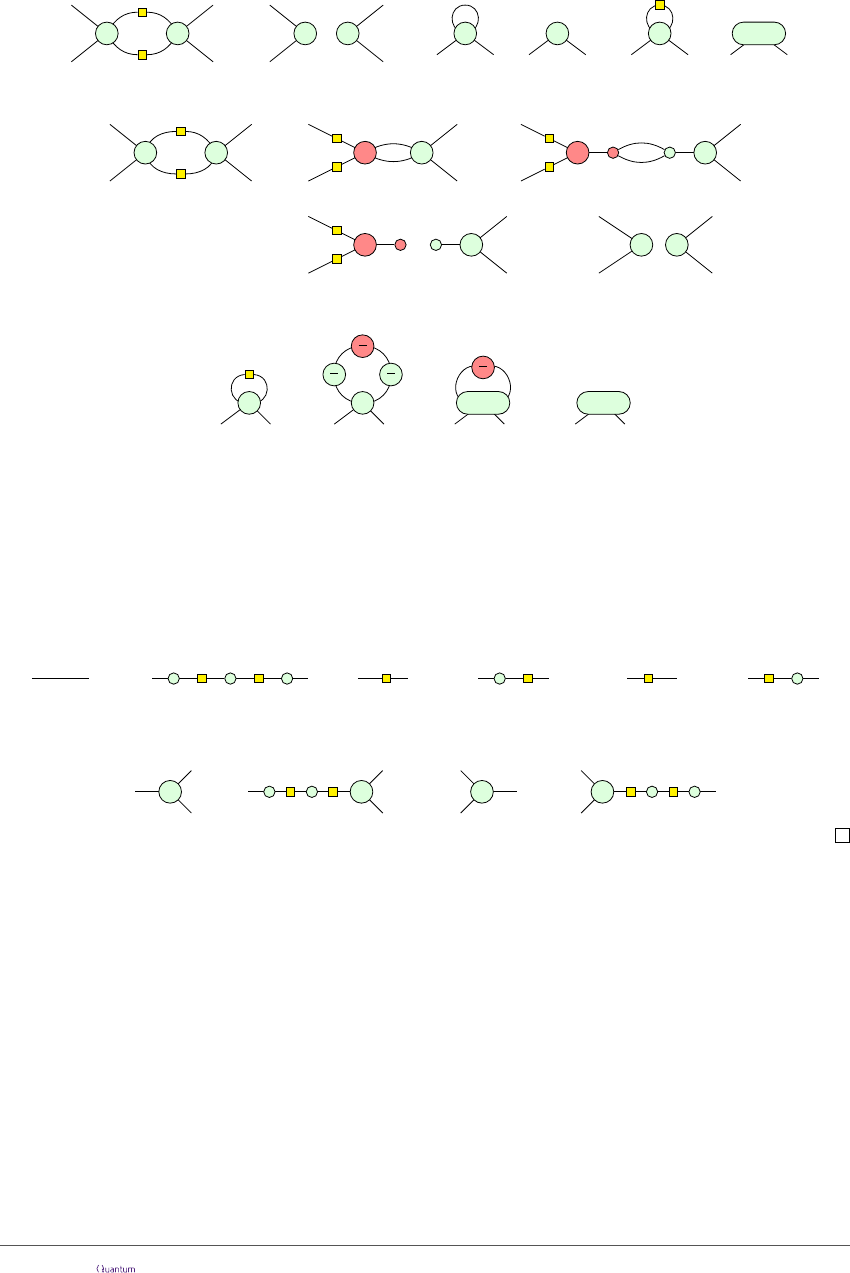Proof. Starting with an arbitrary ZX-diagram, we apply (h) to turn all red spiders into
green spiders surrounded by Hadamard gates. We then remove excess Hadamards via
(i2). Any non-Hadamard edge is then removed by fusing the adjacent spiders with (f ).
Any parallel Hadamard edges or self-loops can be removed via the following 3 rules:
α
β
... ...
=
α
β
... ...
α
...
=
...
α α
...
=
...
α + π
(3)
The ﬁrst one of these follows by using (a):
α
β
... ...
=
α
...
β
...
(h)
=
(f)
α
... ...
β
=
(a)
α
... ...
β
=
(f)
α
... ...
β
(h)
The second one follows by applying (i1) from right to left and then using (f ). For the
last one we do:
α
...
π
2
π
2
α
...
π
2
=
π
2
...
α + π
=
...
α + π
=
(f)
Here, the ﬁrst step is simply the deﬁnition of the Hadamard box, and in the last step we
use the antipode rule (a) and implicitly drop the scalar X-spider that we are left with.
At this point, the ﬁrst 3 conditions are satisﬁed. To satisfy condition 4, we must
deal with two special cases: (a) inputs/outputs not connected to any Z-spider, and (b)
multiple inputs/outputs connected to the same Z-spider. For case (a), there are only two
possibilities left: either an input and an output are directly connected (i.e. a ‘bare wire’),
or they are connected to a Hadamard gate. These situations can both be removed by
right-to-left applications of (i1) and (i2) as follows:
= =
......
=
......
For case (b), we can again use (i1) and (i2) to introduce ‘dummy’ spiders until each
input/output is connected to a single spider:
==
α
...
α
...
α
...
α
...
Once this is done, the resulting ZX-diagram satisﬁes conditions 1-4.
A useful feature of a graph-like ZX-diagram is that much of its structure is captured
by its underlying open graph.
Deﬁnition 3.3. An open graph is a triple (G, I, O) where G = (V, E) is an undirected
graph, and I V is a set of inputs and O V a set of outputs. For a ZX-diagram D, the
underlying open graph G(D) is the open graph whose vertices are the spiders of D, whose
edges correspond to Hadamard edges, and whose sets I and O are the subsets of spiders
connected to the inputs and outputs of D, respectively.
See Figure 2 for an example of converting a circuit into a graph-like diagram with
Lemma 3.2 and how the associated underlying graph is found.
A graph-like ZX-diagram can be seen as an open graph with an assignment of angles
to each of its vertices. Note that, because of Deﬁnition 3.1, the sets I and O in G(D) will
Accepted in Quantum 2020-04-27, click title to verify 7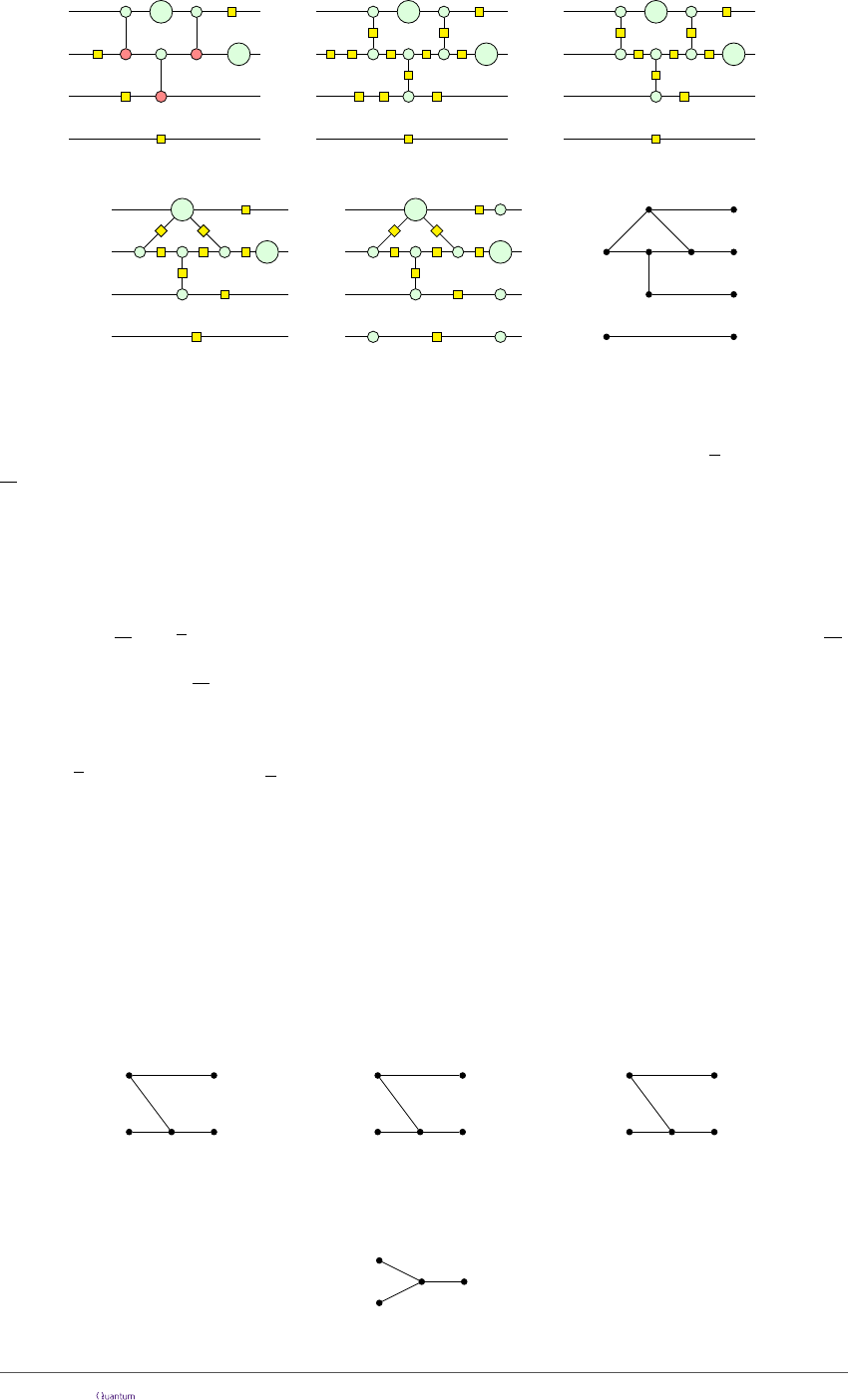α
γ
I 3
I 3
I 3
O
O
O
I 3 O
=
γ
α
=
γ
α
(f) (i1)
α
γ
=
(h)
=
(i2)
α
γ
Figure 2: A circuit, which is transformed into an equivalent graph-like ZX-diagram, and its underlying
open graph.
always be disjoint. When discussing open graphs we will use the notation I := V \ I and
O := V \ O for the non-inputs and non-outputs respectively. The set of neighbours of a
vertex v V will be denoted by N (v).
We are now ready to deﬁne a graph-theoretic condition that will be instrumental in
our circuit extraction procedure.
Deﬁnition 3.4.  Given an open graph G, a focused gFlow (g, ) on G consists of a
function g : O 2
I
and a partial order on the vertices V of G such that for all u O,
1. Odd
G
(g(u)) O = {u}
2. v g(u), u v
where 2
I
is the powerset of I and Odd
G
(A) := {v V (G) | |N (v) A| 1 mod 2} is the
odd neighbourhood of A.
Example 3.5. While not strictly necessary for the following, we brieﬂy give some intuition
for the conditions in Deﬁnition 3.4. They can be understood in a more operational way
with the following game. Suppose we consider an open graph G whose vertices are labelled
with 0’s and 1’s, where a 1 indicates the presence of an error. Deﬁne an operation ﬂip
v
,
which ﬂips all of the bits on the neighbours of a given vertex v. Our goal is to propagate
all of the errors present in G to the outputs using only applications of the operation ﬂip
v
.
For example:
I 3
I 3 O
0
0 01
v
O
1
w
ﬂip
v
I 3
I 3 O
1
0 10
v
O
1
w
ﬂip
w
I 3
I 3 O
0
0 10
v
O
1
w
(4)
For some open graphs and conﬁgurations of errors, this task might be impossible. For
example, there is no solution for the following graph:
I 3
I 3
O
0
0 0
1
Accepted in Quantum 2020-04-27, click title to verify 8However, we can always succeed if we are given the following data: an ordering of
vertices which give a direction of ‘time’ going from inputs to outputs, and, for each vertex,
a correction set g(v) of vertices in the future of v (w.r.t. ) such that applying ﬂip
w
for
all w g(v) ﬂips the bit on v without aﬀecting any other bits, except for those labelling
outputs and g(v) itself. By repeatedly ﬁnding the minimal vertex v (w.r.t. ) with an
error and applying ﬂip
w
to all w g(v), the procedure will eventually propagate all of
the 1’s to the outputs of G.
Remark 3.6. Generalised ﬂow techniques were introduced in the context of measurement-
based quantum computing [13, 14]. Focused gFlow is a special case of the standard notion
of gFlow , where the latter allows other vertices v in the set Odd
G
(g(u)) O, provided
they are in the future of u (i.e. u v). However, as a property of graphs, the two notions
are equivalent: an open graph has a gFlow if and only if it has a focused gFlow. We will
rely on this equivalence in Appendix B.1 to prove the following Lemma.
Lemma 3.7. If D is a graph-like ZX-diagram obtained from a circuit by the procedure
of Lemma 3.2, then G(D) admits a focused gFlow.
Proof. See Appendix B.1.
4 Local complementation and pivoting
Local complementation is a graph transformation introduced by Kotzig .
Deﬁnition 4.1. Let G be a graph and let u be a vertex of G. The local complementation
of G according to u, written as G u, is a graph which has the same vertices as G, but all
the neighbours v, w of u are connected in G u if and only if they are not connected in G.
All other edges are unchanged.
Example 4.2. Local complementations according to the vertices a and b are given by:
G
a
b
d
c
G a
a
b
d
c
(G a) b
a
b
d
c
A related graph-theoretic operation is pivoting, which takes place at a pair of adjacent
vertices.
Deﬁnition 4.3. Let G be a graph, and let u and v be a pair of connected vertices in G.
The pivot of G along the edge uv is the graph G uv := G u v u.
On a graph, pivoting consists in exchanging u and v, and complementing the edges
between three particular subsets of the vertices: the common neighbourhood of u and v
(i.e. N
G
(u) N
G
(v)), the exclusive neighbourhood of u (i.e. N
G
(u) \ (N
G
(v) {v})), and
exclusive neighbourhood of v (i.e. N
G
(v) \ (N
G
(u) {u})):
G
A
B C
vu
G uv
A
B C
v u
For a more concrete illustration of pivoting see Example 4.4 or Equation (6) below.
Accepted in Quantum 2020-04-27, click title to verify 9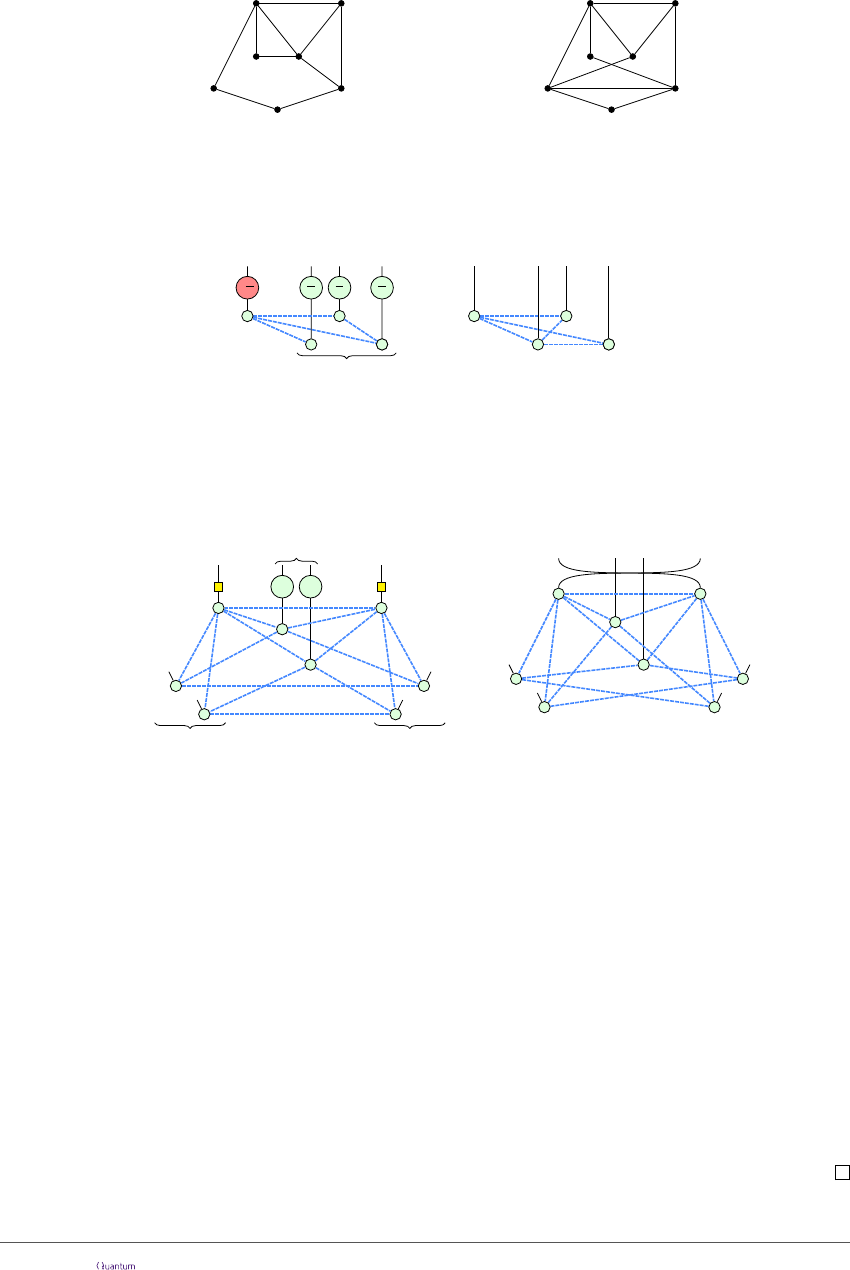Example 4.4. In the graph G below, {a, b} is in the neighbourhood of u alone, {d} is in
the neighbourhood of v alone, and {c} is in the the neighbourhood of both. To perform
the pivot along uv, we complement the edges connecting {a, b} to {d}, {d} to {c} and
{a, b} to {c}. We then swap u and v:
G
u v
d
b
c
a
e
G uv
v u
d
b
c
a
e
Our primary interest in local complementation and pivoting is that each corresponds
to a transformation of ZX-diagrams. In the special case where a ZX-diagram D is a graph-
state, it is possible to obtain a diagram D
0
where G(D
0
) = G(D) u by applying an X
π/2
gate on the spider corresponding to u and Z
π/2
on all of the spiders in N(v):
-
π
2
π
2
π
2
π
2
N(u)
u
=
... ...
(5)
This is a well-known property of graph states, and a derivation using the rules of the
ZX-calculus was given in Ref. . Similarly, it was shown in Ref.  that a pivot
G(D
0
) = G(D) uv can be introduced by applying Hadamard gates on u and v and Z
π
gates on N(u) N(v):
......
ππ
...
... ...
......
...
u v
N(u)\(N(v) {v}) N(v)\(N(u) {u})
N(u) N(v)
=
(6)
Note that the swap on the RHS comes from the vertices u and v being interchanged by
the pivot.
The following theorem will be crucial for our extraction routine. It shows that the
existence of a focused gFlow is preserved by local complementation (resp. pivoting) followed
by the deletion of the vertex (resp. the pair of vertices) on which the transformation is
applied:
Theorem 4.5. Let (G, I, O) be an open graph that admits a focused gFlow, then (G
0
, I, O)
also admits a gFlow in the following two cases:
1. for u / I O, setting G
0
:= (G u)\{u}
2. for adjacent u, v / I O, setting G
0
:= (G uv)\{u, v}
where G \W is the graph obtained by deleting the vertices in W and any incident edges.
Proof. The two cases are proved in Appendix B.2, Lemmas B.5 and B.8 respectively.
Accepted in Quantum 2020-04-27, click title to verify 10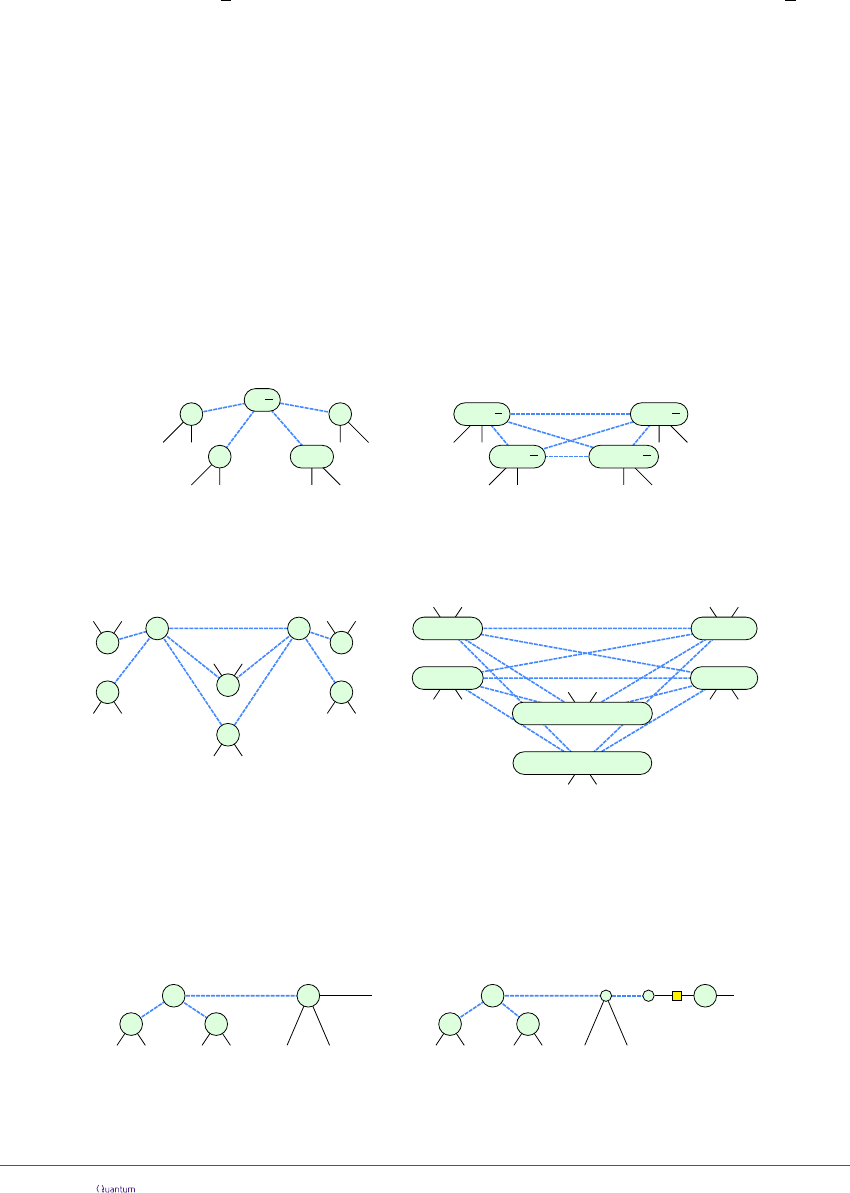5 A simpliﬁcation strategy for circuits
We now have all the necessary machinery to introduce our simpliﬁcation routine. The
general idea is to use local complementation and pivoting based rewrite rules to remove as
many interior spiders as possible. A spider is called interior when it is not connected to
an input or an output, otherwise it is called a boundary spider.
Deﬁnition 5.1. We call a spider Pauli if its phase is a multiple of π, and Cliﬀord if its
phase is a multiple of
π
2
. If the phase of a Cliﬀord spider is an odd multiple of
π
2
(and
hence non-Pauli), we call this a proper Cliﬀord spider.
The graph-like ZX-diagram resulting from the translation of a Cliﬀord circuit contains only
Cliﬀord spiders, since the only time the phase changes on a spider is during a spider-fusion
step, in which case the phases are added together.
We will show that our procedure is able to eliminate all interior proper Cliﬀord spiders
and all Pauli spiders adjacent either to a boundary spider or any interior Pauli spider. In
particular, for Cliﬀord circuits, we will obtain a pseudo-normal form which contains no
interior spiders (cf. Section 7).
The main rewrite rules we use are based on local complementation and pivoting. The
ﬁrst one allows us to remove any interior proper Cliﬀord spider while the second one
removes any two connected interior Pauli spiders.
Lemma 5.2. The following rule holds in the ZX-calculus:
±
π
2
α
1
α
n
...
... ...
=
...
α
1
π
2
...
α
n
π
2
α
2
...
α
n1
...
α
2
π
2
...
α
n1
π
2
...
...
(7)
where the RHS is obtained from the LHS by performing a local complementation at the
marked vertex, removing it, and updating the phases as shown.
Lemma 5.3. The following rule holds in the ZX-calculus:
jπ
α
1
=
α
n
β
1
β
n
γ
1
γ
n
...
...
...
α
n
+ kπ
β
n
+ (j + k + 1)π
...
β
1
+ (j + k + 1)π
γ
1
+ jπ
α
1
+ kπ
...
...
γ
n
+ jπ
...
...
...
...
...
...
...
...
...
...
...
...
(8)
where the RHS is obtained from the LHS by performing a pivot at the marked vertices,
removing them, and updating the phases as shown.
The proofs of these lemmas can be found in Appendix B.3.
We can additionally apply (8) to remove an interior Pauli spider that is adjacent to a
boundary spider. To do this, we ﬁrst turn the boundary spider into a (phaseless) interior
spider via the following transformation, which follows from the rules (f ), (i1), and (i2):
jπ
α
1
=
α
n
α
...
...... ...
u
jπ
α
1
α
n
...
...... ...
α
v w
u
v
(9)
After this transformation, we can perform (8) on the two spiders labelled {u, v} to remove
them. For our purposes, we can then treat w as a boundary spider, and save the single-
qubit unitaries to the right of w separately.
Accepted in Quantum 2020-04-27, click title to verify 11